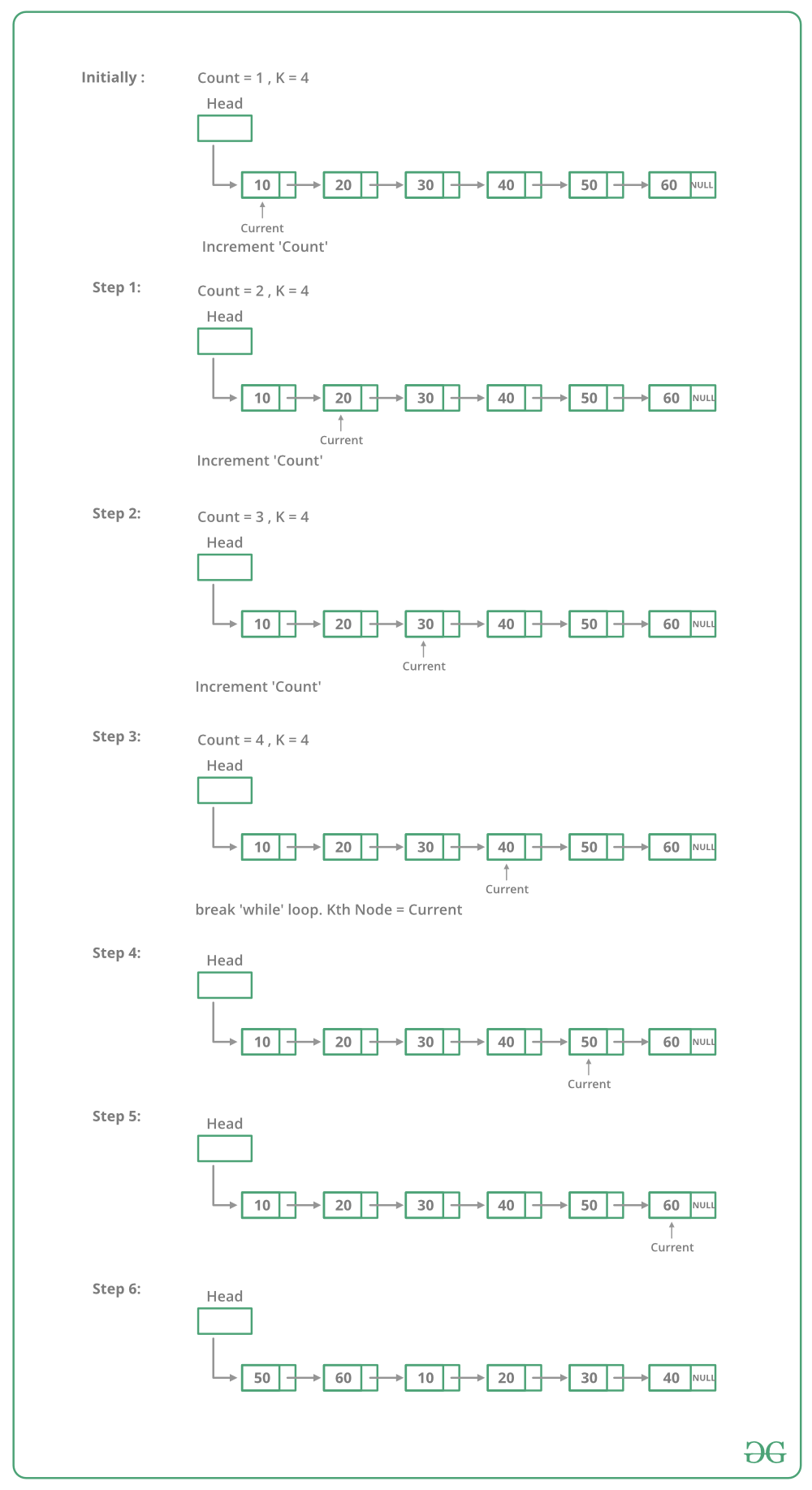# Javascript Program For Rotating A Linked List

• Last Updated : 27 Dec, 2021

Given a singly linked list, rotate the linked list counter-clockwise by k nodes. Where k is a given positive integer. For example, if the given linked list is 10->20->30->40->50->60 and k is 4, the list should be modified to 50->60->10->20->30->40. Assume that k is smaller than the count of nodes in a linked list.

Method 1:
To rotate the linked list, we need to change the next of kth node to NULL, the next of the last node to the previous head node, and finally, change the head to (k+1)th node. So we need to get hold of three nodes: kth node, (k+1)th node, and last node.
Traverse the list from the beginning and stop at kth node. Store pointer to kth node. We can get (k+1)th node using kthNode->next. Keep traversing till the end and store a pointer to the last node also. Finally, change pointers as stated above.

Below image shows how to rotate function works in the code :## Javascript

 ``

Output:

```Given linked list
10  20  30  40  50  60
50  60  10  20  30  40```

Time Complexity: O(n) where n is the number of nodes in Linked List. The code traverses the linked list only once.

Method 2:
To rotate a linked list by k, we can first make the linked list circular and then moving k-1 steps forward from head node, making (k-1)th node’s next to null and make kth node as head.

## Javascript

 ``

Output:

```Given linked list
10 20 30 40 50 60